# C语言学习笔记

C 语言是一种通用的、面向过程式的计算机程序设计语言。

## 指针与字符串

### Part 1. 取地址运算

#### 1. sizyof；

sizeof(int);                       sizeof(i);

	int a = 6;
printf("sizeof(int) = %ld\n",sizeof(int));
printf("sizeof(int) = %ld\n",sizeof(a));
//输出结果均为4;


#### 2.运算符 &；

int a;
printf("%p\n",&a);
printf("%p\n",a);
printf("%p\n",&a);
//将输出三个相同值
printf("%p\n",&a);
//一般相邻地址间距是4；


### Part 2. 指针

#### 1. 指针

• 指针的定义：类型名 *指针变量名（合法的标识符）；
int *p;							/*定义一个指针变量p，指向整型变量*/
double *dp1, *dp2;				/*定义两个指针变量dp1和dp2，指向双精度型变量*/


• 指针变量的赋值：
p = &i;
p = 0;
p = NULL;
p = (int*)1732;		//P指向地址为1732的int型变量。


（1）int *p; p是指针变量
（2）指针变量的数据类型是它所指向的变量的类型，一般情况下一旦指针变量的类型被确定后，它只能指向 同种类型 的变量。

#### 2. 单目运算符 *

• 定义指针变量时被使用，说明被定义的那个变量是指针；
• 用来访问指针的值所表示的地址上的变量；
• 可以做左值也可以做右值；
• int k = *p;
• *p = k + 1;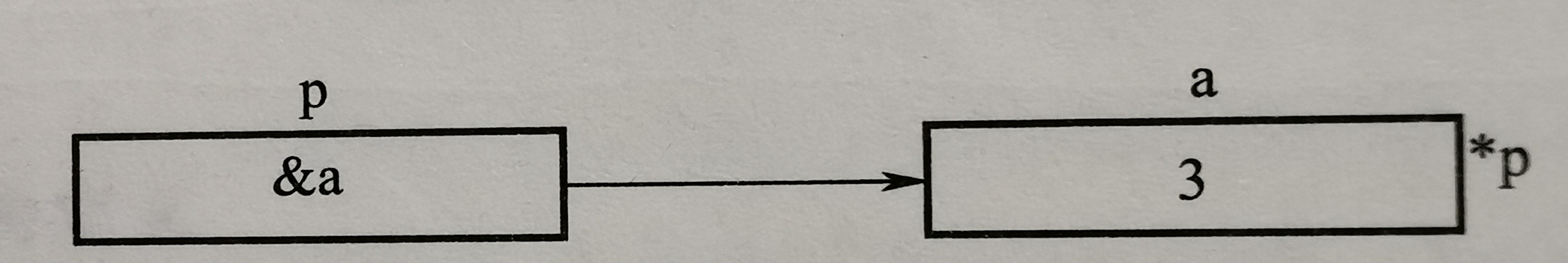#### 3. 指针的使用

• 函数返回多个值，某些值就只能通过指针返回；
• 传入的参数实际上是需要保存带回的结果变量；
• 先定义并赋值再引用；
• 常见错误：定义了指针变量，还没有指向任何变量，就开始使用指针。
int a;
int *p1 = &a;			/*在定义指针p1的同时时给其赋值，使指针p1指向变量a*/
int *p2 = p1;			/*在定义指针p2的同时时给其赋值，使p2和p1值相同*/


#### MOOC中出现的代码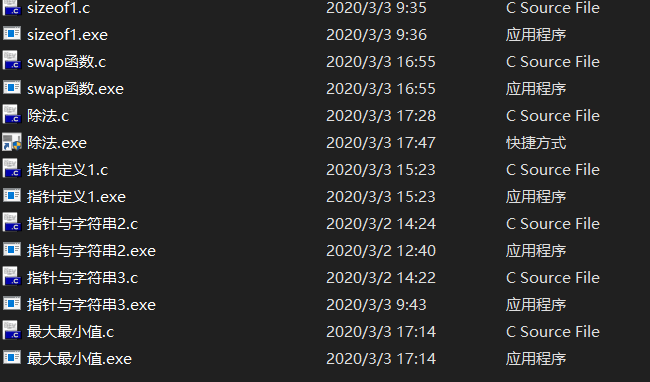#### 个人笔记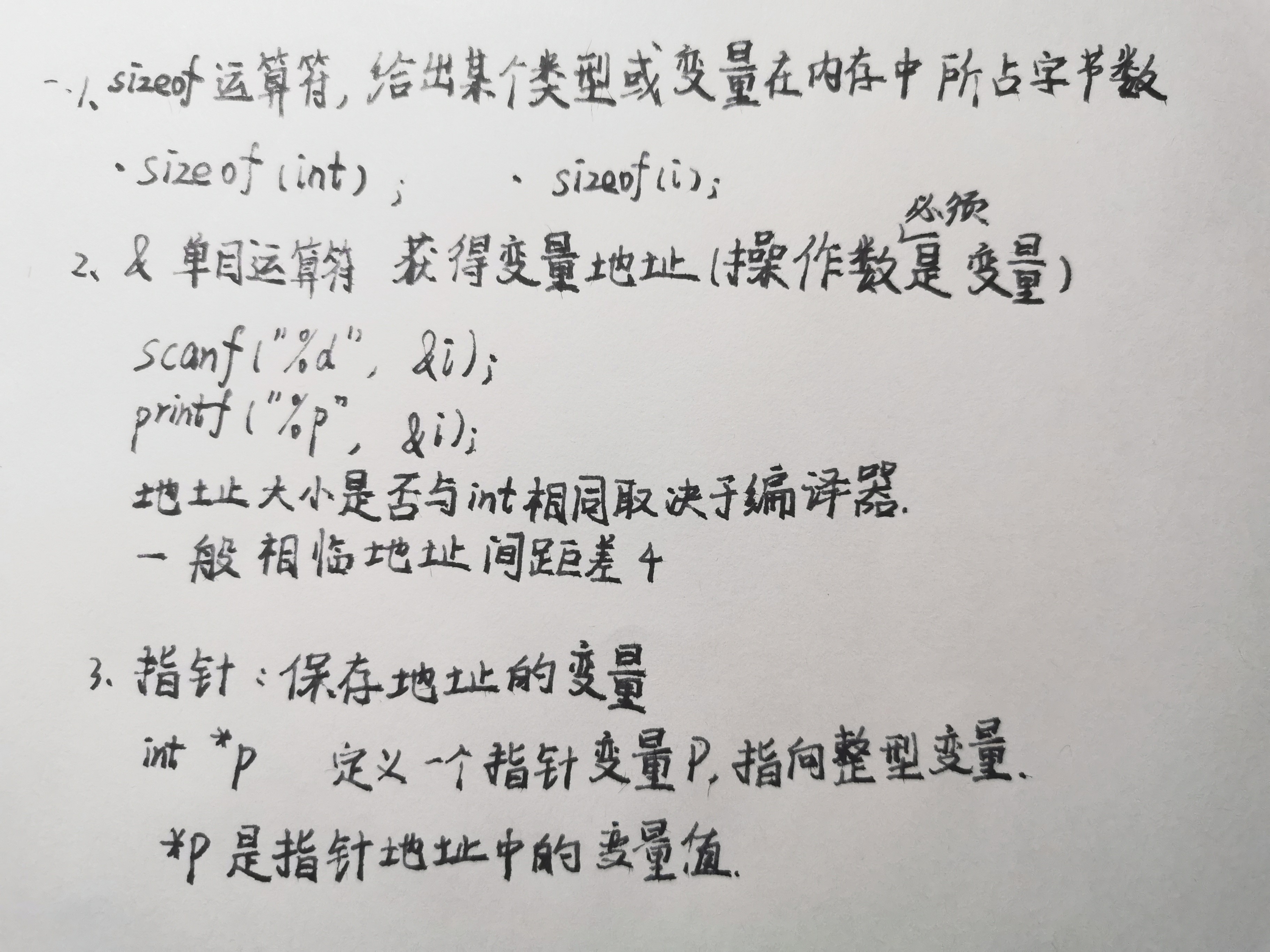#### PTA 练习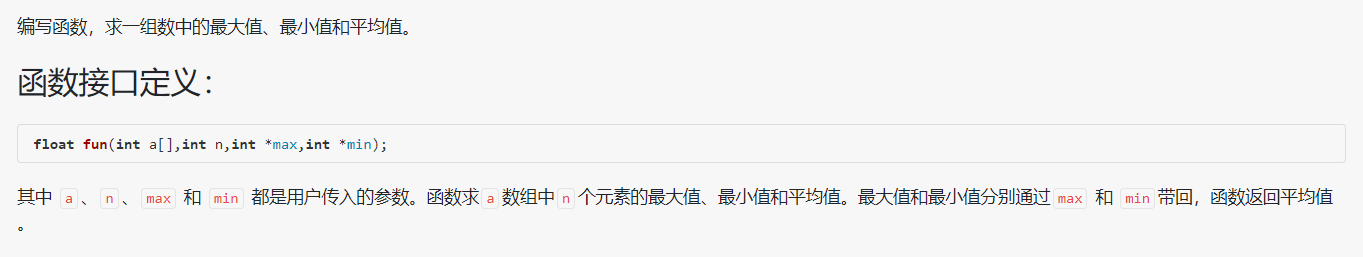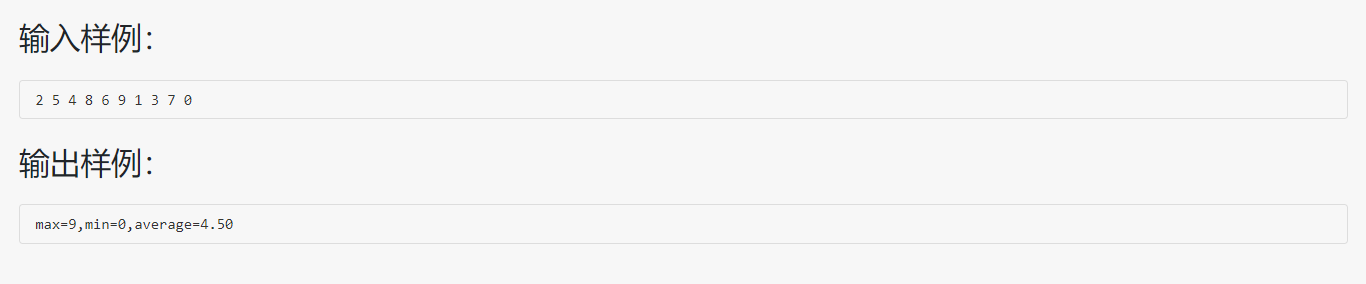#include <stdio.h>
float fun(int a[],int n,int *max,int *min);		     /*函数类型为浮点型，即返回值为浮点型*/
int main()
{
int x,i,m,n;
float p;
for(i=0;i<10;i++)
scanf("%d",&x[i]);
p=fun(x,10,&m,&n);
printf("max=%d,min=%d,average=%.2f\n",m,n,p);	         /*输出结果p为函数返回值*/
return 0;
}

float fun(int a[],int n,int *max,int *min)
{
*min = *max = a;
int i;
float sum = 0;
for(i = 0; i < n; i++)
{
if(a[i] > *max)
*max = a[i];
if(a[i] < *min)
*min = a[i];
sum += a[i];
}

return sum/n;
}

//为了使 sum/n（函数返回值） 为浮点型数 因此要将sum定义为浮点型。


#### 问题及解决

• 输出格式控制问题。
解决方案：查数书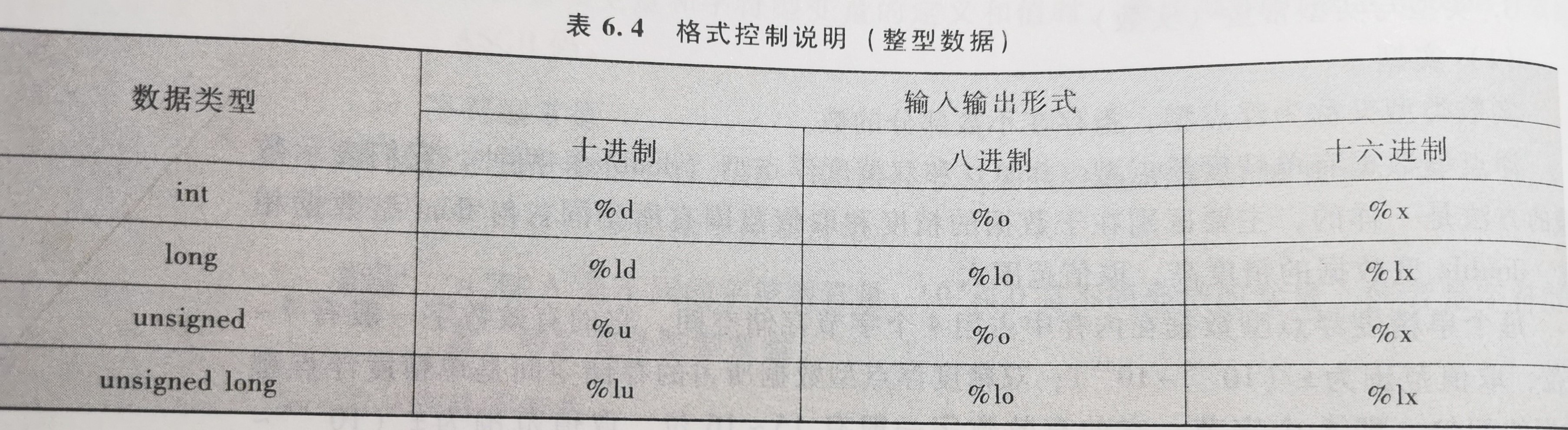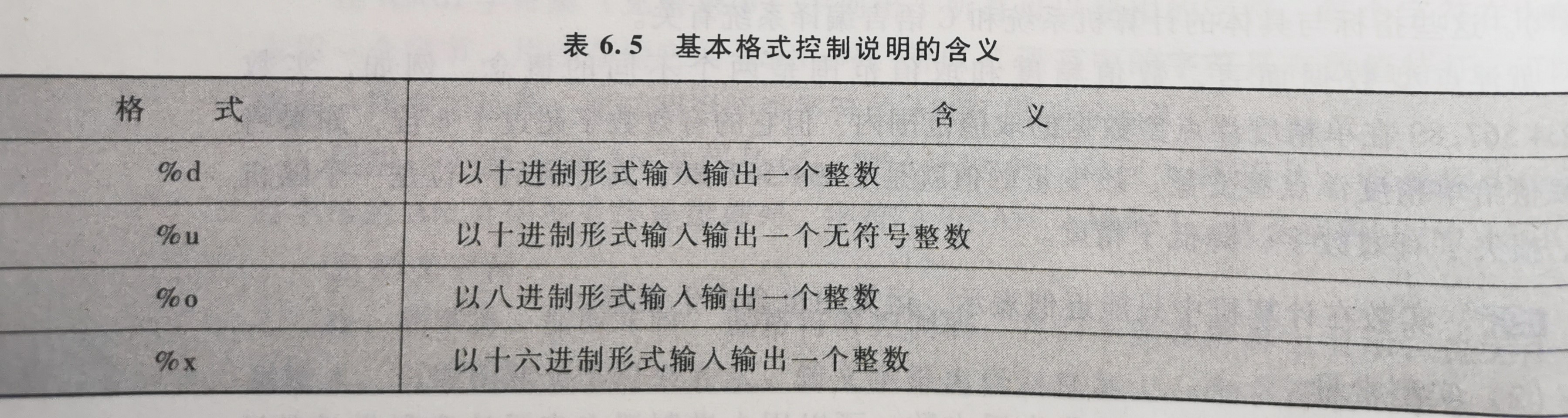• MOOC中最大最小值问题
#include<stdio.h>

void minmax(int a[], int len, int *max, int *min);                    /*用len记录记录数组长度*/

int main(void)
{
int a[] = {1,2,3,4,5,6,7,8,9,11,12,13,14,16,17,21,23,55,};
int min, max;
minmax(a, sizeof(a)/sizeof(a), &min, &max);                 /*sizeof(a)/sizeof(a)确定数组长度*/
printf("min = %d, max = %d\n", min, max);

return 0;
}
void minmax( int a[], int len, int *min, int *max)
{
int i;
*min = *max = a;
for(i = 1; i < len; i++){
if(a[i] < *min){
*min = a[i];
}
if(a[i] > *max){
*max = a[i];
}
}
}
// 函数参数表里的数组实际上是指针，但是可以用数组的运算符[]进行运算。
// 在子函数中，sizeof(a) == sizeof(int *) ；
//这就是为什么函数参数表里要用另外一个变量len记录数组长度，而不能直接用sizeof(a) / sizeof(a)计算。

posted @ 2020-03-03 13:45  ABClazyboy  阅读(256)  评论(1编辑  收藏  举报